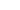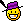Santa Cruz V10.4 carbon weight 14.56kg

• + 4christiaan (Apr 18, 2014 at 13:26)
Looking great mate
• + 1furman1990 (Apr 18, 2014 at 13:27)
Thank You• + 2booostin (Apr 19, 2014 at 18:58)
weight? and where do you get that rad sticker for the 40?
• + 1furman1990 (Apr 20, 2014 at 0:43)
Weight is unknown at this stagestickers for my bike
nldesigns.eu
• + 1furman1990 (May 12, 2014 at 10:30)
just weighted the bike on 2 different scales and its 32 pounds or 14.56kg
• + 2Xgreygoose (Apr 18, 2014 at 18:04)
Sick!! Great job mate :-)
• + 2furman1990 (Apr 19, 2014 at 4:52)
Can't wait to ride it now
• + 2furman1990 (Apr 19, 2014 at 4:54)
• + 1Xgreygoose (Apr 19, 2014 at 5:54)
• + 1furman1990 (Apr 19, 2014 at 5:57)
my lunch brake isn't long enough to describe it haha
• + 2furman1990 (Apr 28, 2014 at 1:07)
I'll set up my BOS VOID today to the settings you have sent me
• + 2Xgreygoose (Apr 28, 2014 at 4:45)
Let me know how it go's
• + 2furman1990 (Apr 30, 2014 at 10:13)
Hey man I've tried your settings and works very good thanks• + 2Xgreygoose (Apr 30, 2014 at 10:58)
Nice one!
• + 1arquitetodh (May 26, 2014 at 13:31)
Amazing bike! What about NW rimg?
• + 1furman1990 (May 26, 2014 at 13:38)
Thank you, since I have it never dropped the chain• + 2mtbmedia123 (Apr 19, 2014 at 23:51)
Where is the DVO???• + 2furman1990 (Apr 20, 2014 at 0:40)
I'm not really a fan of DVO and especially it's price man
• + 1furman1990 (Apr 23, 2014 at 3:24)
Anyone have a problem with Shimano Saint rattling brake pads ?
• + 2jujubiker01 (Apr 19, 2014 at 8:55)
Really really nice !
• + 1furman1990 (Apr 19, 2014 at 12:03)
cheers man
• + 2arquitetodh (Apr 18, 2014 at 14:31)
• + 1furman1990 (Apr 19, 2014 at 4:53)
Thanks I appreciate it
• + 2Stuuriaan (Apr 19, 2014 at 0:05)
Pimpin'!• + 1furman1990 (Apr 19, 2014 at 4:51)
Haha thanks